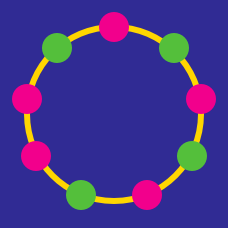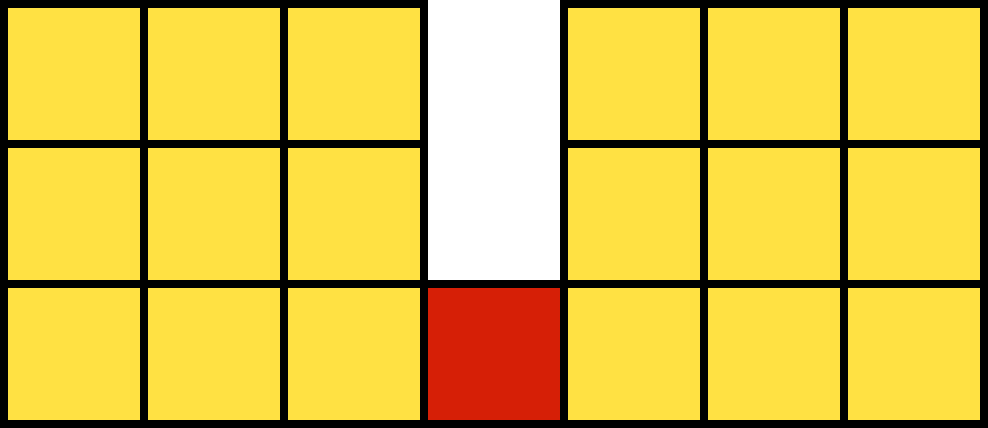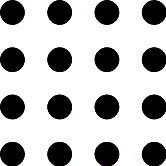Probability

# Discrete Mathematics Warmups: Level 3 ChallengesThe figure above consists of 19 unit squares. How many rectangles are there in the figure?


Note: Squares are also considered as rectangles.

$\large \displaystyle S = \{{1,2,3,\ldots, 19,20}\}$

How many subsets of three numbers each can be formed from the set above so that no two consecutive numbers are in the set?

Three married couples arrange a party. They arrive at the party one at a time, the couples not necessarily arriving together. They all, upon arriving, shake the hand of everyone already there, except their own spouse. When everyone has arrived, someone asks all the others how many hands they shook upon arriving, and gets five different answers. How many hands did he himself shake upon arriving?In the $4 \times 4$ grid, how many squares have all 4 vertices on these dots?

Bonus: Generalize this for $n \times n$ grid.

Two distinct integers are selected at random from 1, 2, 3, ... , 100 and multiplied with each other. Find the probability (to two decimal places) that the product is divisible by 3.

×